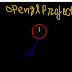### Create Pendulum in OpenGL Computer Graphics ProgramWe have created many simple programs using OpenGL C/C++ in this blog. In this post we are going to create another simple opengl program. We are creating a simple pendulum. You can use these code as base to create a project particlualry based on the use of pendulum.
Introduction
A pendulum can be define as a certain mass of object tied from a pivot that can swings back and forth due to force of gravity. The Pendulum swing at the same distance in both the directions. The angle made by pendulum from the centre on bothe side are equal and called amplitude of pendulum.

Design and Code
This simple pendulum opengl graphics program we are going to code will have two things - circle and lines. We are going to create a simple circle as clock and attache thread to it with a small circle to it. We will swing the circle right and left from the center point of it attachement with thread.

First in this computer graphics program we are going to write a function for coding circles. We are goint to name this functions as drawcircle. The function will have four arguments segments, radius, x, y. As name suggest these are angle (segments), radius of thread, x and y coordinates.

float drawCircle(float segments,float radius,float sx,float sy)
{
glBegin(GL_LINE_LOOP);
for(int i=0;i<segments;i++)
{
float theta=2.0*3.142*float(i)/float(segments); //get the current angle
glVertex2f(x+sx,y+sy);
}
glEnd();
}

Now we are going to draw the pendulum with the help of draw functions which looks like -

void draw(float x1,float y1,float angle)
{
float segments=100;

//Drawing Clock main Circle
glLineWidth(4);
glColor3f(1,0,0);

//Drawing Minute Line
glColor3f(1,1,0);
glLineWidth(2);
glBegin(GL_LINES);
glVertex2f(x1,y1);
glEnd();

//Drawing Hour Line
glColor3f(1,0,0);
glLineWidth(2);
glBegin(GL_LINES);
glVertex2f(x1,y1);
glEnd();
//Drawing Pendulum Circle

glColor3f(0,0,1);
//glLineWidth(2);

glBegin(GL_LINES);
glVertex2f(x2,y2);
glEnd();
}

In above opengl computer graphics program code we have drawn a simple clock with hour and minute hands.You may skip therse as they are just to make this program look realstic and more sensible. In last few line we were able to draw the pendulum circle and the thread with the help of circle functions above and GL_LINES.

As we have the draw function we applying the below logic to make the right and left movment of pendulum and thread.

if(angle>315)
{
angle=315;
inc=-inc;
}
if(angle<225)
{
angle=225;
inc=-inc;
}

We are going to place all the code in display function and then create the main functions. You can see the output below.
Outpu Pendulum OpenGL Program

Projects Suggestion
1. Simple Old Mechanical Clock
2. Clock tower with long pendulam Clock
3. Different kinds of swings
4. Circus swings with jokers
5. Amusment park with swings
Get the full code of the